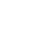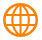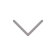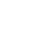﻿ Understand the rated current of the cable in three minutes－OMG EV CableWebsite### FAQ

##### Home > News > FAQ
Understand the rated current of the cable in three minutes

Date：2018/5/3 15:36:13

The working temperature is 30℃, and the current-carrying capacity under long-term continuous 90% load is as follows:
1.5mm2-13A
2.5mm2-26A
4mm2-32A
6 mm2-47A
16mm2-92A
25mm2-120A
35mm2-150A

2
Current conversion power:
1A=220W,
10A=2200W, and so on.
For example: if the current carrying capacity is 14A copper wire, that is: 220W×14=3080W, then the power of 1.5 square copper wire is 3.08 kW.
Long-term current allowed by the national standard:
4 square is 25-32A
6 square is 32-40A
These are theoretical safety values, and the limit values ​​must be greater than these.
The maximum power allowed for 2,5 square copper wire is 5500W.

4 square 8000W, 6 square 9000W is no problem.
40A digital electric meter is normal, 9000W is no problem. The mechanical 12000W will not burn out.

3

Copper core wire allows long-term current:
2.5 square millimeters (16A～25A)
4 square millimeters (25A～32A)
6 square millimeters (32A～40A)
for example :
1. The power consumption of each computer is about 200~300W (about 1~1.5A), then 10 computers need a 2.5 square millimeter copper core wire for power supply, otherwise, fire may occur.
2. The power consumption of the three large air conditioners is about 3000W (about 14A), then a single 2.5-mm-square copper core wire is required for one air conditioner.
3. The current house incoming line is generally 4 square millimeters of copper wire. Therefore, household appliances that are turned on at the same time must not exceed 25A (that is, 5500 watts). It is useless to replace the wires in the house with 6 square millimeters of copper wire. , Because the wire that enters the meter is 4 square millimeters.
4. Early housing (15 years ago) The incoming line is generally 2.5 square millimeters of aluminum wire. Therefore, household appliances that are turned on at the same time must not exceed 13A (that is, 2800 watts).
5. Household appliances with large power consumption are air conditioner 5A (1.2 hp), electric water heater 10A, microwave oven 4A, rice cooker 4A, dishwasher 8A, washing machine with drying function 10A, electric water heater 4A.
In the fire caused by the power supply, 90% is caused by the heating of the joints, so all the joints must be welded, and the contact devices that cannot be welded must be replaced within 5 to 10 years (such as sockets, air switches, etc.).

4

Copper core wire and cable current carrying capacity standard cable current carrying capacity decision
Multiply by 2.5 and multiply by nine.
Thirty-five times three and a half, both points are reduced by five.
Conditions are subject to change and conversion, and high-temperature 10% copper upgrade.
The number of pipes to be worn is two to three, four to eight, seventy-six percent full load.
Description:
This formula does not directly indicate the current-carrying capacity (safe current) of various insulated wires (rubber and plastic insulated wires) but is expressed by "cross-section multiplied by a certain multiple" and obtained by mental calculation.
"2.5 times multiply by nine, minus one by one," refers to various cross-section aluminum core insulated wires of 2.5mm2 and below, and its current carrying capacity is about 9 times the number of cross-sections. Such as 2.5mm2 wire, the current-carrying capacity is 2.5 × 9 = 22.5 (A). The multiple relationships between the current carrying capacity of 4mm2 and above conductors and the number of cross-sections are to go up along the line number, and the multiple is reduced by 1, ie 4×8, 6×7, 10×6, 16×5, 25×4.

"Thirty-five times three-five, two pairs of two points minus five", said that the current-carrying capacity of 35mm2 wire is 3.5 times the number of cross-sections, which is 35 × 3.5 = 122.5 (A). From the conductor of 50mm2 and above, the multiple relationships between the current-carrying capacity and the number of cross-sections become a group of two two-wire numbers, and the multiple is reduced by 0.5 in turn. That is, the current-carrying capacity of the 50 and 70mm2 conductors is three times the number of cross-sections; the current-carrying capacity of the 95 and 120mm2 conductors is 2.5 times its cross-sectional area, and so on.

"Conditions are subject to change and conversion, and high-temperature 10% copper upgrade". The above formula is determined by copper core insulated wire and exposed laying at an ambient temperature of 25℃. If the aluminum core insulated wire is laid in an area where the ambient temperature is longer than 25℃ for a long time, the current-carrying capacity of the wire can be calculated according to the above formula, and then it can be discounted by 10%; when using aluminum wire instead of aluminum wire, Its current-carrying capacity is slightly larger than that of aluminum wire of the same specification. You can calculate the current-carrying capacity that is one more wire number than the aluminum wire according to the above formula. For example, the current car